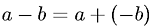Equations > Algebra > Addition/Subtraction > Definition of Subtraction

### Definition of SubtractionLatex Code:

MathML Code:

 $a-b=a+\left(-b\right)$

MathType 5.0: